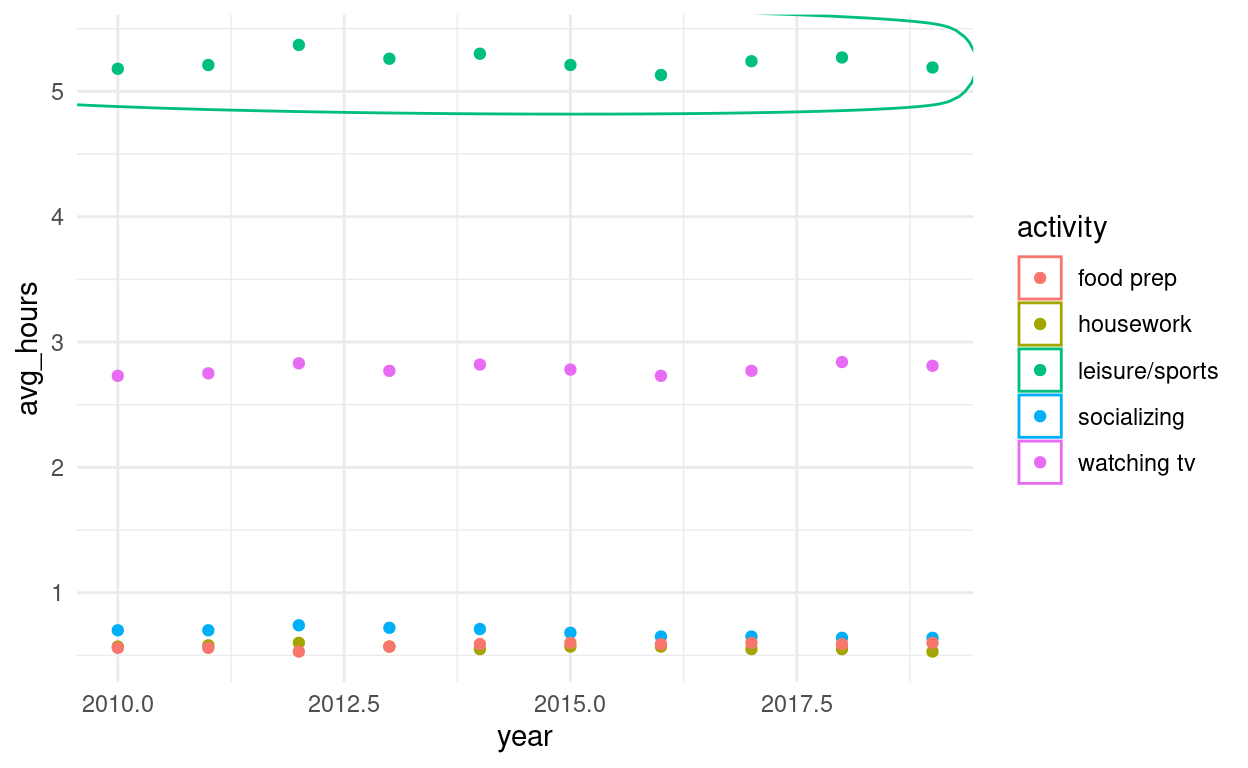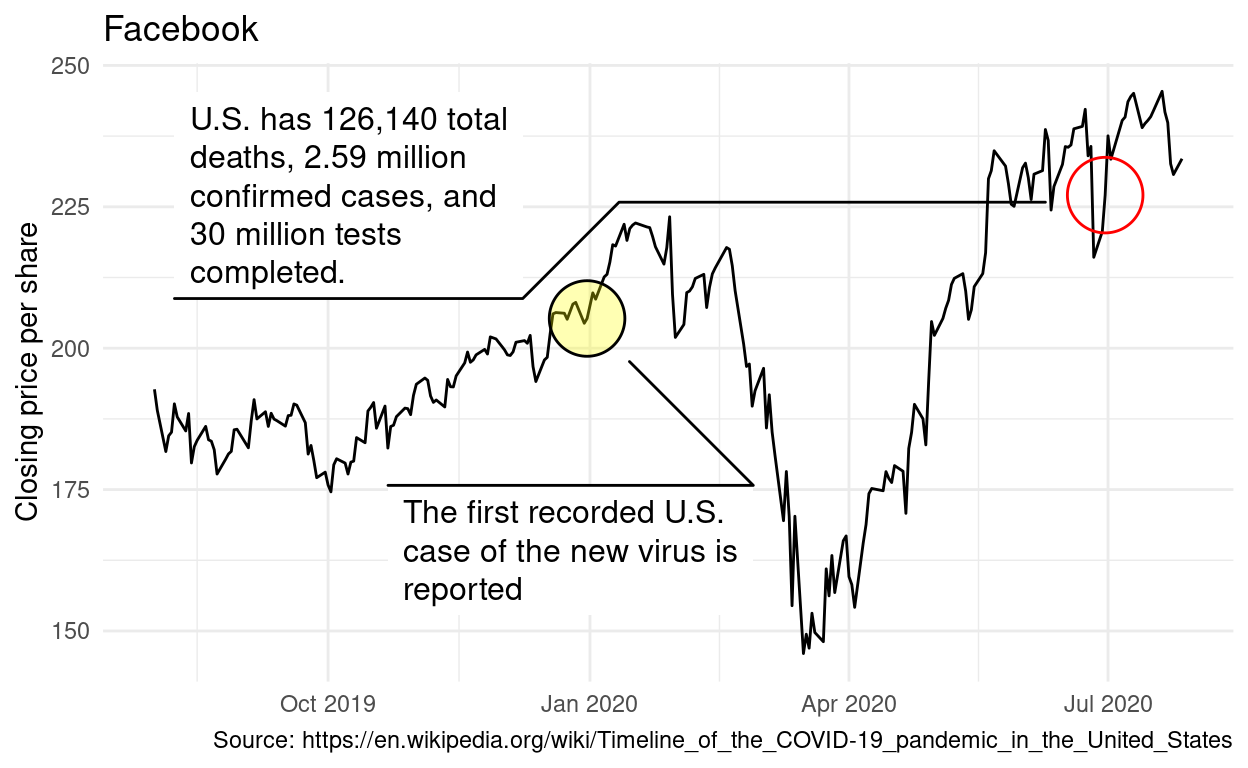# Data Visualization

Code for Quiz 9.

1. Load the R package we will use.
``````library(tidyverse)
library(echarts4r)
library(ggforce) #install  before using for the first time
library(tidyquant)  #install  before using for the first time
theme_set(theme_minimal()) # set all of the plot themes
``````
1. Quiz questions
• Replace all the instances of ‘SEE QUIZ’. These are inputs from your moodle quiz.
• Replace all the instances of ‘???’. These are answers on your moodle quiz.
• Run all the individual code chunks to make sure the answers in this file correspond with your quiz answers
• After you check all your code chunks run then you can knit it. It won’t knit until the ??? are replaced
• The quiz assumes that you have watched the videos, downloaded (to your examples folder) and worked through the exercises in exercises_slides-73-108.Rmd.

# Question: e_charts-1

Create a bar chart that shows the average hours Americans spend on five activities by year. Use the `timeline` argument to create an animation that will animate through the years.

• `spend_time` contains 10 years of data on how many hours Americans spend each day on 5 activities
• read it into `spend_time`
``````spend_time  <- read_csv("https://estanny.com/static/week8/spend_time.csv")
``````

e_charts-1

Start with `spend_time`

• THEN `group_by` year
• THEN create an `e_chart` that assigns activity to the x-axis and will show activity by year (the variable that you grouped the data on)
• THEN use `e_timeline_opts` to set autoPlay to TRUE
• THEN use `e_bar` to represent the variable `avg_hours` with a bar chart
• THEN use `e_title` to set the main title to ‘Average hours Americans spend per day on each activity’
• THEN remove the legend with `e_legend`
``````spend_time  %>%
group_by(year)  %>%
e_charts(x =activity , timeline = TRUE) %>%
e_timeline_opts(autoPlay = TRUE)  %>%
e_bar(serie = avg_hours)  %>%
e_title(text ='Average hours Americans spend per day on each activity')  %>%
e_legend(show = FALSE )
``````

# Question: echarts-2

Create a line chart for the activities that American spend time on. Start with `spend_time`

• THEN use `mutate` to convert year from an number to a string (year-month-day) using mutate
• first convert year to a string “201X-12-31” using the function `paste`
• paste will paste each year to 12 and 31 (separated by -)
• THEN use `mutate` to convert year from a character object to a date object using the `ymd` function from the lubridate package (part of the tidyverse, but not automatically loaded). `ymd` converts dates stored as characters to date objects.
• THEN `group_by` the variable activity (to get a line for each activity)
• THEN initiate an `e_charts` object with year on the x-axis
• THEN use `e_line` to add a line to the variable `avg_hours`
• THEN add a tooltip with `e_tooltip`
• THEN use `e_title` to set the main title to ‘Average hours Americans spend per day on each activity’
• THEN use `e_legend`(top = 40) to move the legend down (from the top)
``````spend_time  %>%
mutate(year = paste(year, "12","31", sep = "-"))  %>%
mutate (year = lubridate::ymd(year))  %>%
group_by(activity)  %>%
e_charts(x  = year)  %>%
e_line(serie = avg_hours)  %>%
e_tooltip()  %>%
e_title(text = 'Average hours Americans spend per day on each activity')  %>%
e_legend(top = 40)
``````

# Question - modify slide 82

• Create a plot with the `spend_time` data
• assign `year` to the x-axis
• assign `avg_hours` to the y-axis
• assign `activity` to color
• ADD points with `geom_point`
• ADD `geom_mark_ellipse`
• filter on activity == “leisure/sports”
• description is “Americans spend the most time on leisure/sport”
``````ggplot(spend_time, aes(x = year, y = avg_hours, color = activity,)) +
geom_point() +
geom_mark_ellipse(aes(filter = activity == "leisure/sports",
description = "Americans spend on average more time each day on leisure/sports than the other activities"))
``````# Question: tidyquant

Modify the tidyquant example in the video

Retrieve stock price for Facebook, ticker: FB, using `tq_get`

• from 2019-08-01 to 2020-07-28
• assign output to df
``````df  <-tq_get("FB", get = "stock.prices",
from = "2019-08-01", to = "2020-07-28" )
``````

Create a plot with the `df` data

• assign `date` to the x-axis
• assign `close` to the y-axis
• ADD a line with with `geom_line`
• ADD `geom_mark_ellipse`
• filter on a date to mark. Pick a date after looking at the line plot. Include the date in your Rmd code chunk.
• include a description of something that happened on that date from the pandemic timeline. Include the description in your Rmd code chunk
• fill the ellipse yellow
• ADD `geom_mark_ellipse`
• filter on the date that had the minimum `close` price. Include the date in your Rmd code chunk.
• include a description of something that happened on that date from the pandemic timeline. Include the description in your Rmd code chunk
• color the ellipse red
• ADD `labs`
``````ggplot(df, aes(x = date, y = close)) +
geom_line() +
geom_mark_ellipse(aes(
filter  = date == "2019-12-31",
description = "The first recorded U.S. case of the new virus is reported"
), fill  = "yellow",) +
geom_mark_ellipse(aes(
filter  = date == "2020-06-30",
description = "U.S. has 126,140 total deaths, 2.59 million confirmed cases, and 30 million tests completed."
), color = "red", ) +
labs(``````  ggsave(filename = "preview.png",